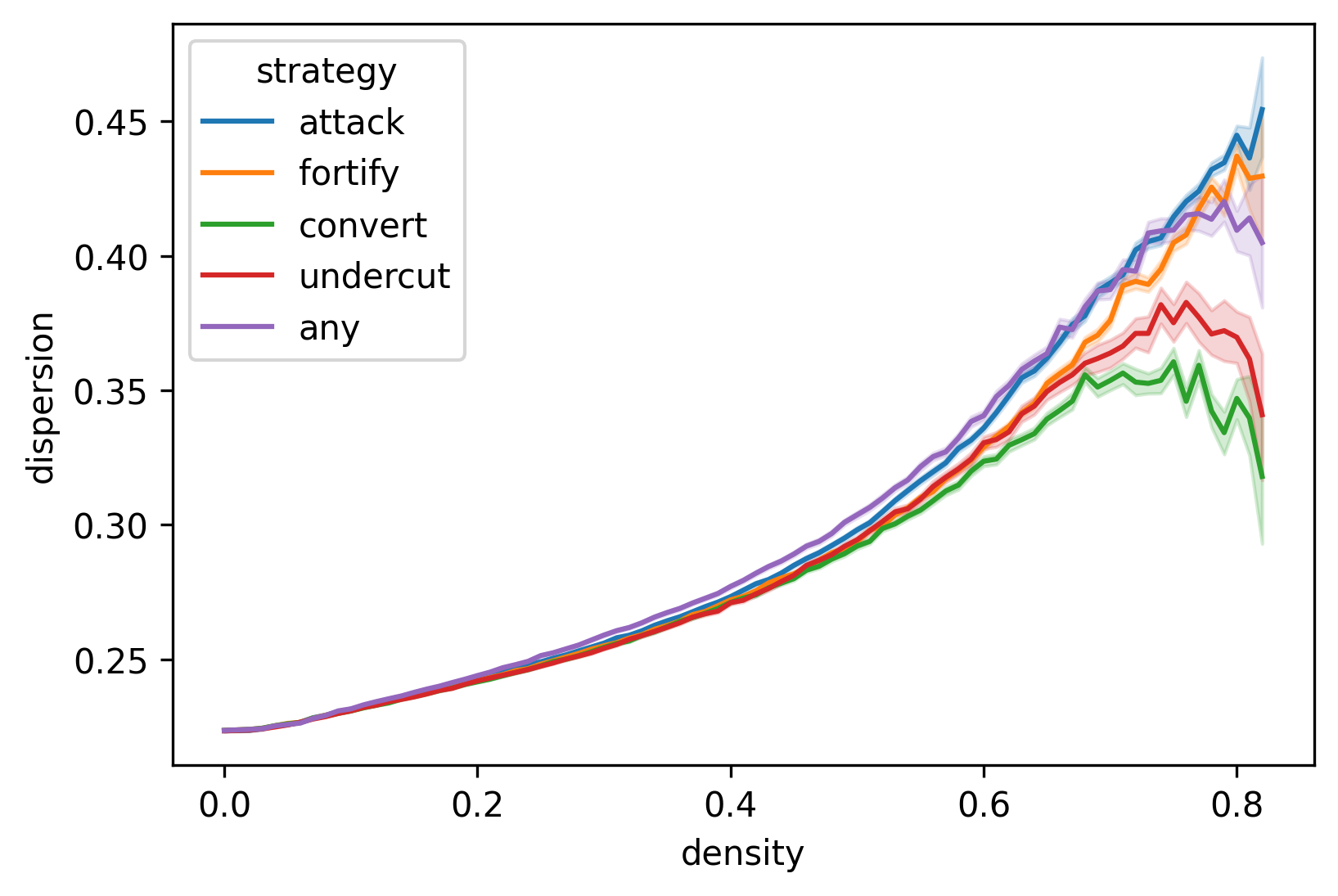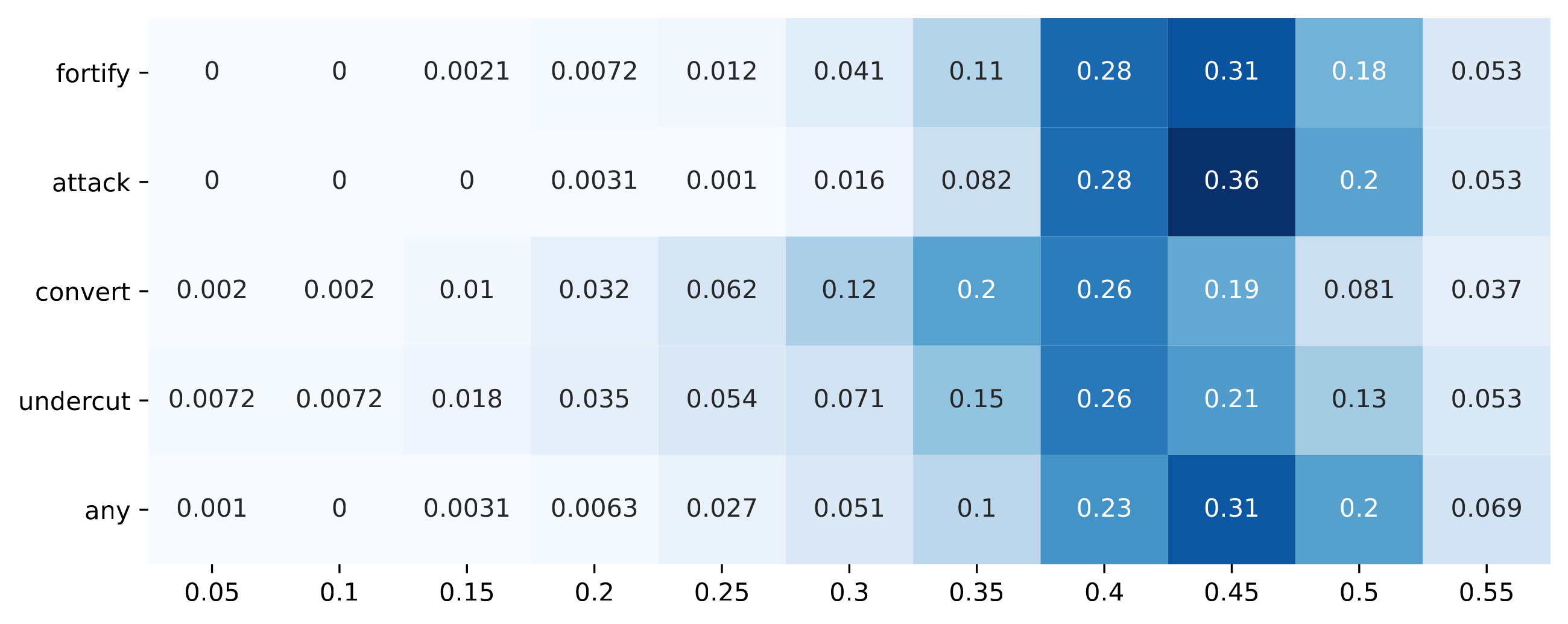### Correction 1

Figure 1 replaces the original Figure 3 in Section 4.7 in Kopecky (2022) as the original version reported results from incorrect measurement. In the original manuscript, the dispersion measurement was normalised incorrectly to a [0, 0.5] range. This was due to a factor 2 missing from Definition 1 in Section 4.6 of the original manuscript. Adding this factor to the definition yields measurements normalised to a [0, 1] range. The correct calculation for dispersion is as follows:

 $\text{dispersion}(\tau) := 2\Bigg(\frac{1}{N}\sum_{i\in A_\tau}^{N}\Bigg(\frac{1}{N-1}\sum_{j\neq i}^{N-1} \delta(i,j) - \underbrace{\frac{1}{\left|{A_\tau\choose 2}\right|} \sum_{(x,y)\in{A_\tau}} \delta(x,y)}_{\text{mean distance (constant)}} \Bigg)^2\,\Bigg)^{1/2}$Figure 1. Mean dispersion plotted against density and depending on argumentation strategy in the main experiment. Shaded areas show confidence intervals based on statistical bootstrapping.

This implies that all dispersion measurements in the original manuscript must be doubled. The new figure shows that dispersion values are closer to the observed group divergence values than reported in the original manuscript. Comments made in the original article about dispersion values being at a lower level were biased by the erroneous normalisation. Indeed, dispersion appears to be a useful approximation of group divergence as it does not require community structuring.

However, as the findings of the original manuscript relied mainly on group divergence and group consensus measurements, the main conclusions of the original article are unaffected by this error, and even strengthened by the correct normalisation of dispersion values.

### Correction 2

Figure 2 replaces the original Figure 13 included in the original Appendix C. Figure 2 contains raw dispersion values calculated with the correct normalisation.Figure 2. Histogram of dispersion values at the first debate stage of each simulation run that passes a density of 0.8, interpreted as a heat map. For every $$i$$ on the $$x$$ axis, the area assigned to it shows values in the half-open interval $$[i-1, i]$$. For example, the tick 0.25 shows the proportion of observed values in the interval $$[0.2,0.25]$$ relative to all observed values of the same strategy. Since there are 1,000 simulation runs in each strategy, absolute values can be easily obtained from the relative values.

### Correction 3

The model code in the original article at this link: https://doi.org/10.5281/zenodo.5067834 has been updated with a new pairwise_dispersion() function. The normalisation has been corrected from taupy version 0.5 onwards.

The author would like to apologise for any inconvenience caused.

### References

KOPECKY, F. (2022). Arguments as Drivers of Issue Polarisation in Debates Among Artificial Agents, Journal of Artificial Societies and Social Simulation. 25 (1) 4: http://jasss.soc.surrey.ac.uk/25/1/4.html. [doi:10.18564/jasss.4767]